Courses

# Match the Following, Integer Value, of Electromagnetic Induction and Alternating Current, Past year JEE Notes | EduRev

## Class 12 Physics 35 Years JEE Mains &Advance Past year Paper

Created by: Abhishek Kapoor

## JEE : Match the Following, Integer Value, of Electromagnetic Induction and Alternating Current, Past year JEE Notes | EduRev

The document Match the Following, Integer Value, of Electromagnetic Induction and Alternating Current, Past year JEE Notes | EduRev is a part of the JEE Course Class 12 Physics 35 Years JEE Mains &Advance Past year Paper.
All you need of JEE at this link: JEE

Match the Following

DIRECTIONS : Each question contains statements given in two columns, which have to be matched.

The statements in Column-I are labelled A, B, C and D, while the statements in Column-II are labelled p, q, r and s. Any given statement in Column-I can have correct matching with ONE OR MORE statement(s) in Column-II. The appropriate bubbles corresponding to the answers to these questions have to be darkened as illustrated in the following example :

If the correct matches are A-p, s and t; B-q and r; C-p and q; and D-s then the correct darkening of bubbles will look like the given.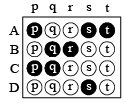Q.1. You are given many resistances, capacitors and inductors. These are connected to a variable DC voltage source (the first two circuits) or an AC voltage source of 50 Hz frequency (the next three circuits) in different ways as shown in Column II. When a current I (steady state for DC or rms for AC) flows through the circuit, the corresponding voltage V1 and V2 , (indicated in circuits) are related as shown in Column I. Match the two

 Column I Column II (A) I ≠ 0,V1 isproportional  to I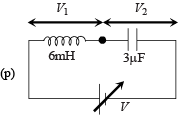(B) I ≠ 0, V2>V1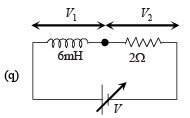(C) V1 = 0,V2 = V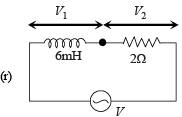(D) I ≠ 0,V2 isproportional to I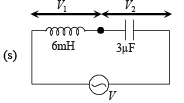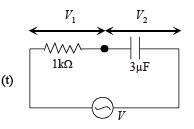Ans. A-r, s, t; B-q, r, s, t; C-p, q; D-q, r, s, t

Solution. The following are the important concepts which are applied in the given situation.

(i) For DC circuit, in steady state, the current I through the capacitor is zero. In case of L-C circuit, the potential difference across the inductor is zero and that across the capacitor is equal to the applied potential difference.

In case of L-R circuit, the potential difference across inductor is zero across resistor is equal to the applied voltage.
(ii) For AC circuit in steady state, Irms curr ent flows through the capacitor, inductor and resistor. The potential difference across resistor, inductor and capacitor is proportional to I.
(iii) For DC circuit, for changing current, the potential difference across inductor, capacitor or resistor is proportional to the current.

Integer Value Correct Type

Q.1. A series R-C combination is connected to an AC voltage of angular frequency ω = 500 radian/s. If the impedance of the R-C circuit is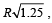the time constant (in millisecond) of the circuit is    (2011)

Ans. 4

Solution. Time constant = RC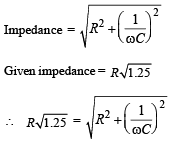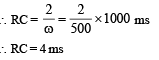Q.2. A circular wire loop of radius R is placed in the x-y plane centered at the origin O. A square loop of side a(a<<R) having two turns is placed with its centre at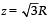along the axis of the circular wire loop, as shown in figure. The plane of the square loop makes an angle of 45° with respect to the z-axis. If the mutual inductance between the loops is given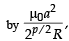then the value of p is     (2012)

Ans. 7

Solution. The magnetic field due to current carrying wire at the location of square loop is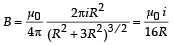The mutual induction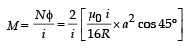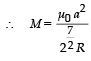Q.3. Two inductors L1 (inductance 1 mH, internal resistance 3 Ω) and L2 (inductance 2 mH, internal resistance 4 Ω), and a resistor R (resistance 12 Ω) are all connected in parallel across a 5 V battery. The circuit is switched on at time t = 0.

The ratio of the maximum to the minimum current (Imax / I min) drawn from the battery is      (JEE Adv. 2016)

Ans. 8

Solution.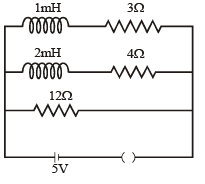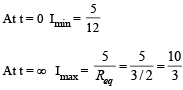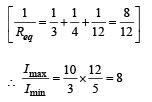Offer running on EduRev: Apply code STAYHOME200 to get INR 200 off on our premium plan EduRev Infinity!

42 docs|19 tests

,

,

,

,

,

,

,

,

,

,

,

,

,

,

,

,

,

,

,

,

,

,

,

,

,

,

,

,

,

,

;